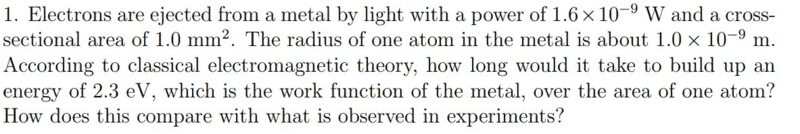# How to approach this photoelectric effect question

• Safder Aree

#### Safder Aree

I am currently taking my first Quantum Mechanics course and was given this problem in a practice set(we are supposed to refer to old intro textbooks). We haven't covered the photoelectric effect (just theory) much in class and reading through other textbooks, I wasn't able to find any similar questions. Any hints on how to approach this question would be greatly appreciated. Thank you.

1. Homework Statement
https://i.imgur.com/1FT6skF.jpgKE = hf - phi

## The Attempt at a Solution

I know phi, so KE = hf - 2.3eV
However, I do not know how to find f since I don't know wave length.
I also do not understand how I can relate it back to time.

#### Attachments

Last edited by a moderator:

This problem is asking you to perform a classical calculation. That means that you should do it without recourse to the photoelectric formula which, as you already found out, is not useful here. You know the incident power, so find how long it will take for 2.3 eV to be collected.

This problem is asking you to perform a classical calculation. That means that you should do it without recourse to the photoelectric formula which, as you already found out, is not useful here. You know the incident power, so find how long it will take for 2.3 eV to be collected.

Would this just be P = W/T? Then solving for T in this instance?

It would be that. Be careful how you use the numbers and watch the units that are given to you.

It would be that. Be careful how you use the numbers and watch the units that are given to you.
So
2.3ev in Joules = 3.68501*10^-19

1.6*10^-9 = W/T
T = 1.6*10^9/3.68501*10^-19
T=2.303*10^-10

The units seems to work out if I did dimensional analysis right. If this right?

Also, please put units next to numbers. What kind of units should W have in the equation P = W/T? What kind of units does 1.6×10-9 have as given in the question?

•Safder Aree
Also, please put units next to numbers. What kind of units should W have in the equation P = W/T? What kind of units does 1.6×10-9 have as given in the question?

Okay I'll write in the units.

So Power is in watts (J/S)

P = 1.6*10^-9 J/s
W= 2.3eV = 3.68501*10^-19 Joules

Therefore,

P= W/T
T = W/P

T = (1.6*10^9 J/s) /(3.68501*10^-19 J)
T=2.303*10^-10s

That's the time required for the entire area to collect 2.3 eV because P is the area of the cathode. Is that the time that the question is asking you to find?

•Safder Aree
That's the time required for the entire area to collect 2.3 eV because P is the area of the cathode. Is that the time that the question is asking you to find?

No it's asking for it over the area of one atom. So this is my strategy to find that:

Find the area of the atom (assuming 2d dimensions):
A= pi*r^2
A = 3.14159*10^-18m^2

Then multiply the time by the ratio of A/Total Area:

=2.303*10^-10s * (3.14159*10^-18 m^2)/(1*10^-3 m^2)
=7.235*10^-25

No it's asking for it over the area of one atom. So this is my strategy to find that:

Find the area of the atom (assuming 2d dimensions):
A= pi*r^2
A = 3.14159*10^-18m^2

Then multiply the time by the ratio of A/Total Area:

=2.303*10^-10s * (3.14159*10^-18 m^2)/(1*10^-3 m^2)
=7.235*10^-25
I assume your numbers are given in seconds. Please avoid calculating numbers without trying to figure out if your answers make sense. Here is a question I have: If it takes T=2.303*10^-10s for the entire area to collect 2.3 eV, does it make sense that an atom, which has a much much smaller area, will collect the same amount of energy in only 7.235*10^-25 s?

I assume your numbers are given in seconds. Please avoid calculating numbers without trying to figure out if your answers make sense. Here is a question I have: If it takes T=2.303*10^-10s for the entire area to collect 2.3 eV, does it make sense that an atom, which has a much much smaller area, will collect the same amount of energy in only 7.235*10^-25 s?

You're right, it doesn't make sense it takes less time to collect more energy with less surface area. Would swapping the ratio be the correct method? So taking Total Area/area of the atom?

What do you think? Don't guess, but reason it out. If the total area of 1 mm2 receives 1.6×10-9 W, what fraction of that power does one atom receive given the radius of one atom?

What do you think? Don't guess, but reason it out. If the total area of 1 mm2 receives 1.6×10-9 W, what fraction of that power does one atom receive given the radius of one atom?

This makes sense now to me. I was confused about the how to approach it. Thank you for all the help!